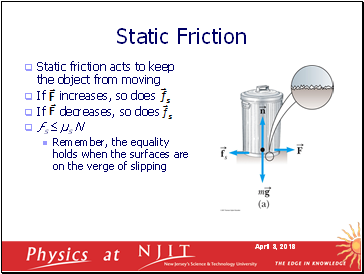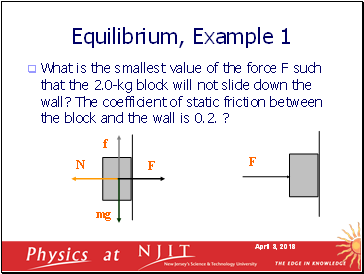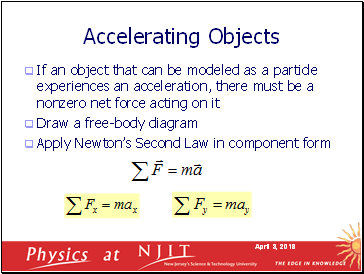# Mechanics LecturePage 2

#### WATCH ALL SLIDES

Magnitude: Friction is proportional to the normal force

Static friction: Ff = F  μsN

Kinetic friction: Ff = μkN

μ is the coefficient of friction

The coefficients of friction are nearly independent of the area of contact (why?)

Forces of Friction: Magnitude

Slide 9## Static Friction

Static friction acts to keep the object from moving

If increases, so does

If decreases, so does

ƒs  µs N

Remember, the equality holds when the surfaces are on the verge of slipping

Slide 10## Kinetic Friction

The force of kinetic friction acts when the object is in motion

Although µk can vary with speed, we shall neglect any such variations

ƒk = µk N

Slide 11## Explore Forces of Friction

Vary the applied force

Note the value of the frictional force

Compare the values

Note what happens when the can starts to move

Slide 12## Hints for Problem-Solving

Read the problem carefully at least once

Draw a picture of the system, identify the object of primary interest, and indicate forces with arrows

Label each force in the picture in a way that will bring to mind what physical quantity the label stands for (e.g., T for tension)

Draw a free-body diagram of the object of interest, based on the labeled picture. If additional objects are involved, draw separate free-body diagram for them

Choose a convenient coordinate system for each object

Apply Newton’s second law. The x- and y-components of Newton second law should be taken from the vector equation and written individually. This often results in two equations and two unknowns

Solve for the desired unknown quantity, and substitute the numbers

Slide 13## Objects in Equilibrium

Objects that are either at rest or moving with constant velocity are said to be in equilibrium

Acceleration of an object can be modeled as zero:

Mathematically, the net force acting on the object is zero

Equivalent to the set of component equations given by

Slide 14What is the smallest value of the force F such that the 2.0-kg block will not slide down the wall? The coefficient of static friction between the block and the wall is 0.2. ?

Equilibrium, Example 1

F

Slide 15Go to page:
1  2  3  4  5